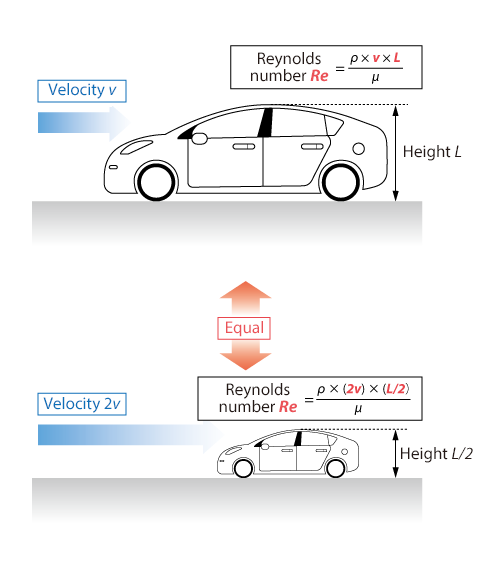#1. TOP
2. Resource Library
3. CAE Glossary
4. P-T index
5. Reynolds' law of similarity

# Reynolds' law of similarity

## Reynolds' law of similarity

Reynolds' Law of Similarity states that when there are two geometrically similar flows, both are essentially equal to each other, as long as they embrace the same Reynolds number.

Let us take an example of an actual vehicle and a half scale model as shown in the following diagram. The Reynolds numbers of both agree when the velocity of the half scale model is doubled. In this state, the proportions of viscous force and inertia force of both cases are equal; hence, the surrounding flows can be defined as similar.